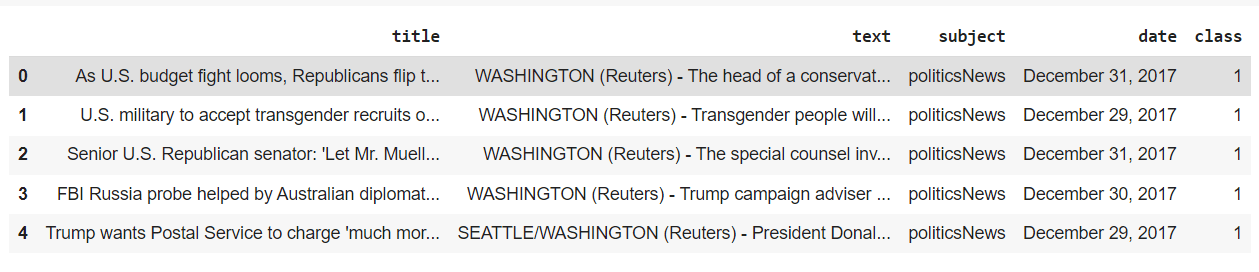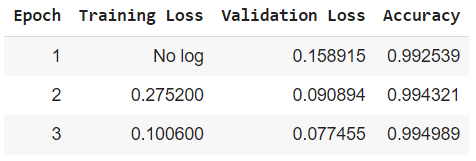In this tutorial, you’ll learn how to do text classification with GPT-J Transformer. GPT-J is the open-source alternative to OpenAI’s GPT-3.

As an example, we’ll attempt to detect fake news from text. We walked you through a nice rundown of Hugging Face Pipelines last week so we’ll continue with that by using the Hugging Face implementation of the GPT-J transformer.

Transformer architecture is a type of deep learning architecture that learns text representations using self-attention mechanism. Transformers are the state-of-the-art Natural Language Processing (NLP) models that achieve best performance on many NLP benchmarks.

## Importing Transformers Library

``pip install datasets transformers[sentencepiece]``

## Importing the Dataset

You will be using the Fake and real News Dataset from Kaggle. The dataset consists of two CSV files: True.csv, which contains data for true news, and Fake.csv, which contains data for fake news, according to the dataset.

To be clear, there are concerns with the accuracy of these datasets based on the methodologies used to classify the articles, so I wouldn’t use it to truly sift through modern news articles. Instead, consider this a demonstration of the types of text classification exercises Python machine learning allows you to perform.

The following script imports the two files as Pandas dataframes. A `class` column is added to both the dataframes. The value of the `class` column is 1 for true news, and 0 for fake news. These are actually the values that the GPT-J model will be predicting.

Finally the two dataframes are concatenated to form a single dataset. The output shows that the dataset consists of four columns: title, text, subject, date, and class. We will use the `text` column to train our transformer model.

``````import pandas as pd

ds_true["class"] = 1

ds_fake["class"] = 0

ds_complete =  pd.concat([ds_true, ds_fake], axis=0)

OutputNotice our use of the Pandas concat function to merge our two datasets.

The following script shows the number of true and fake news articles in our dataset (1=> True, 0=> Fake). You can see that the class distribution is almost even.

``ds_complete["class"].value_counts()``

Output

``````0    23481
1    21417
Name: class, dtype: int64``````

We’re now going to use the `Trainer` class from the transformers library which expects the dataset to be in the form of `Dataset`, or `DatasetDic` format.

The following script converts the Pandas dataframe containing the dataset to the `Dataset` class. The script also removes unwanted columns. We’ll keep the `title` column in the dataset, so you’ll be able to use an articles title to detect fake news later.

``````from datasets import Dataset, DatasetDict
dataset = Dataset.from_pandas(ds_complete)
dataset = dataset.remove_columns(['subject', 'date', '__index_level_0__'])
dataset = dataset.shuffle(seed = 555)
dataset``````

Output

``````Dataset({
features: ['title', 'text', 'class'],
num_rows: 44898
})``````

Finally, to train and evaluate our GPT-J transformer model, we’ll divide our dataset into training, validation and test sets.

``````train_testvalid = dataset.train_test_split(test_size=0.4)

test_valid = train_testvalid['test'].train_test_split(test_size=0.5)

dataset = DatasetDict({
'train': train_testvalid['train'],
'test': test_valid['test'],
'valid': test_valid['train']})

dataset``````

Output

``````DatasetDict({
train: Dataset({
features: ['title', 'text', 'class'],
num_rows: 26938
})
test: Dataset({
features: ['title', 'text', 'class'],
num_rows: 8980
})
valid: Dataset({
features: ['title', 'text', 'class'],
num_rows: 8980
})
})``````

## Tokenizing the dataset

Before performing any machine learning operations on our dataset, we need to convert text to its numeric representation before it can be used to train a transformers model.

You can use the `AutoTokenizer` class from the transformer library to convert text to its numeric representation, as required by the corresponding transformer model.

The following script creates a tokenizer for the GPT-J model. You just have to pass the model path to the `AutoTokenizer` class’s `from_pretrained()` method. In the following script we pass the path to the GPT-J transformer. You’re welcome to pass other hugging face transformer models if you want.

``````from transformers import AutoTokenizer, AutoModelForSequenceClassification, DataCollatorWithPadding

model_name = "ydshieh/tiny-random-gptj-for-sequence-classification"

tokenizer = AutoTokenizer.from_pretrained(model_name)

The script below tokenizes our dataset using the tokenizer defined in the previous script.

``````def tokenize_function(examples):

tokenized_datasets = dataset.map(tokenize_function, batched=True)``````

The `Trainer` class expects labels as the column name for the column containing class labels. In our dataset, the class column contains the labels. The following script renames the class column to labels.

``tokenized_datasets = tokenized_datasets.rename_column("class", "labels")``

I put together a Python Developer Kit with over 100 pre-built Python scripts covering data structures, Pandas, NumPy, Seaborn, machine learning, file processing, web scraping and a whole lot more - and I want you to have it for free. Enter your email address below and I'll send a copy your way.

Yes, I'll take a free Python Developer Kit

## Training the Model and Making Predictions

We’re now ready to fine-tune the GPT-J transfomer model on our dataset.

You can import the pretrained weights for the GPT-J transformer model using the `from_pretrained()` method of `AutoModelForSequenceClassification` class. This class basically adds a classification layer on top of your already pretrained model.

``````model = AutoModelForSequenceClassification.from_pretrained(model_name, num_labels=2)

# resize model embedding to match new tokenizer
model.resize_token_embeddings(len(tokenizer))``````

The next step is to define the metrics you want to use for evaluating the model performance. The following script defines a method `compute_metrics()` which uses accuracy as the performance metric for model evaluation.

``````import numpy as np

def compute_metrics(eval_pred):
logits, labels = eval_pred
predictions = np.argmax(logits, axis = -1)
return metric.compute(predictions=predictions, references=labels)``````

Next, you need to pass your training arguments to the `TrainingArguments` class. The next script defines training arguments. Here’s an explanation of what each argument means:

1. `output_dir`: the directory where your model will be saved.
2. `evaluation_strategy`: strategy for evaluating your model performance. Setting it to `epoch` evaluates model performance after each epoch.
3. `fp16`: setting it to true improves model training speed, but it’s only allowed on CUDA devices.
4. `per_device_train/eval_batch_size:` the number of records to use during each training batch.

The default number of epochs is 3. You can change the number of epochs by passing a value for the `num_train_epochs` parameter.

``````from transformers import TrainingArguments, Trainer

training_args = TrainingArguments(output_dir="test_trainer",
evaluation_strategy="epoch",
fp16 = True,
per_device_train_batch_size= 64,
per_device_eval_batch_size= 64
)``````

Finally, you can pass the model, training arguments, training and evaluation datasets, the method for performance evaluation, and the tokenizer to the `Training` class object.

We’ll use our `train` set for training our model and the `valid` set for evaluating our model performance during training.

``````trainer = Trainer(
model=model,
args=training_args,
train_dataset=tokenized_datasets['train'],
eval_dataset=tokenized_datasets['valid'],
compute_metrics=compute_metrics,
tokenizer=tokenizer
)``````

Now you’re ready to fine-tune your GPT-J model. To do so, you just have to call the `train()` method on the `Trainer` class object.

``trainer.train()``

The output below shows that our GPT-J model achieves an accuracy of 99.49% after 3 epochs. Pretty impressive, though this is unusual and is what caused people to question the accuracy of the dataset in classifying real and fake news. We’ll ignore that detail for now.

Output## Making Predictions on Test Set

Once your model is trained, you should evaluate it on a dataset that your model has never seen before. This gives you an estimate of how well your model will perform in the production environment.

You can use the `predict()` method from the `Trainer` class to make predictions on a new dataset. In the following script we make predictions on our test dataset which we did not use during the training.

``predictions = trainer.predict(tokenized_datasets['test'])``

The `predict()` method returns a tuple of three items. The third item of the tuple contains performance metrics.

``predictions``

The output below shows that our model achieves an accuracy of 99.09% on an unseen test set.

Output

``````{'test_loss': 0.0825851783156395,
'test_accuracy': 0.9930957683741648,
'test_runtime': 15.1146,
'test_samples_per_second': 594.127,
'test_steps_per_second': 9.329}``````

Finally, you can also get the individual predictions for each of the records in the test set using the `np.argmax(predictions)` script.

The following script shows how you can evaluate your model performance (e.g. accuracy) using the Python Scikit-learn library, like we did in our sklearn machine learning tutorial.

``````from sklearn.metrics import accuracy_score
print(accuracy_score(np.argmax(predictions, axis=-1), tokenized_datasets['test']['labels']))``````

Output

``0.9930957683741648``